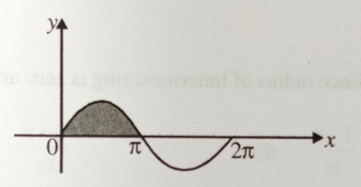Two forces  and  are acting on a particle.

The resultant force acting on particle is:

(A)  $2\stackrel{^}{\mathrm{i}}+5\stackrel{^}{\mathrm{j}}+4\stackrel{^}{\mathrm{k}}$

(B)  $2\stackrel{^}{\mathrm{i}}-5\stackrel{^}{\mathrm{j}}-4\stackrel{^}{\mathrm{k}}$

(C)  $\stackrel{^}{\mathrm{i}}-3\stackrel{^}{\mathrm{j}}-2\stackrel{^}{\mathrm{k}}$

(D)  $\stackrel{^}{\mathrm{i}}-\stackrel{^}{\mathrm{j}}-\stackrel{^}{\mathrm{k}}$

Difficulty Level:

Two forces  and  are acting on a particle.

The angle between  is:

(A)  $\mathrm{\theta }={\mathrm{cos}}^{-1}\left(\frac{3}{2\sqrt{5}}\right)$

(B)  $\mathrm{\theta }={\mathrm{cos}}^{-1}\left(\frac{3}{5\sqrt{2}}\right)$

(C)  $\mathrm{\theta }={\mathrm{cos}}^{-1}\left(\frac{2}{3\sqrt{5}}\right)$

(D)  $\mathrm{\theta }={\mathrm{cos}}^{-1}\left(\frac{\sqrt{3}}{5}\right)$

Concept Questions :-

Resultant of Vectors

Difficulty Level:

Two forces  and  are acting on a particle.

The magnitude of the component of force ${\stackrel{\to }{\mathrm{F}}}_{1}$ along force ${\stackrel{\to }{\mathrm{F}}}_{2}$ is:

(A)  $\frac{5}{6}$

(B)  $\frac{5}{3}$

(C)  $\frac{6}{5}$

(D)  $\frac{5}{2}$

Concept Questions :-

Resultant of Vectors

Difficulty Level:

$\mathrm{A}=4\mathrm{i}+4\mathrm{j}-4\mathrm{k}$ and $\mathrm{B}=3\mathrm{i}+\mathrm{j}+4\mathrm{k}$, then angle between vectors A and B is:

(A)  $180°$

(B)  $90°$

(C)  $45°$

(D)  $0°$

Concept Questions :-

Resultant of Vectors

Difficulty Level:

A curve is governed by the equation y=sinx, then what is the area enclosed by the curve and x-axis between x =0 and x =$\mathrm{\pi }$ is (shaded region)1. 1 units

2. 2 units

3. 3 units

4. 4 units

Concept Questions :-

Integration
High Yielding Test Series + Question Bank - NEET 2020

Difficulty Level:

Calculate the area of disk of radius 'a' using integration

1. $\frac{{\mathrm{\pi a}}^{2}}{2}$

2. ${\mathrm{\pi a}}^{2}$

3. $\frac{3}{2{\mathrm{\pi a}}^{2}}$

4. $2{\mathrm{\pi a}}^{2}$

Concept Questions :-

Integration
High Yielding Test Series + Question Bank - NEET 2020

Difficulty Level:

The acceleration of a particle starting from rest varies with time according to relation, . Find the velocity of the particle at time instant t.

1. $\alpha {t}^{2}+\beta t$

2. $\alpha {t}^{2}+\frac{\beta t}{2}$

3. $\frac{\alpha {t}^{2}}{2}+\beta t$

4. $2\alpha {t}^{2}+\beta t$

Concept Questions :-

Integration
High Yielding Test Series + Question Bank - NEET 2020

Difficulty Level:

The displacement of particle is zero at t=0 and at t=t it is x. It starts moving in the x direction with velocity, which varies as $v=k\sqrt{x}$, where k is constant. The velocity-

1. varies with time

2. is independent to time

3. inversely proportional to time

4. inversely proportional to acceleration

Concept Questions :-

Integration
High Yielding Test Series + Question Bank - NEET 2020

Difficulty Level:

The acceleration of a particle is given as $a=3{x}^{2}$. At t=0, v=0, x=0, the velocity at t =2 sec will be-

1.  0.05 m/s

2. 0.5 m/s

3. 5 m/s

4. 50 m/s

Concept Questions :-

Integration
High Yielding Test Series + Question Bank - NEET 2020

Difficulty Level:

The acceleration of a particle is given by a=3t and at t=0, v=0, x=0. The velocity and displacement at t = 2 sec will be-

1. 6 m/s, 4 m

2. 4 m/s, 6 m

3. 3 m/s, 2 m

4. 2 m/s, 3 m

Concept Questions :-

Integration